# MP Board Class 9th Maths Solutions Chapter 15 Probability Ex 15.1

## MP Board Class 9th Maths Solutions Chapter 15 Probability Ex 15.1

Question 1.
In a cricket match, a batswoman hits a boundary 6 times out of 30 balls she plays. Find the probability that she did not hit a boundary.
Solution:
Total number of trials = 30
Number of balls in which boundary hit = 6
Number of balls in which boundary not hit = 30 – 6 = 24
Let E denotes the event that she did not hit a boundary.
P(E) = $$\frac{24}{30}$$ =0.8.Question 2.
1500 families with 2 children were selected randomly, and the following data were recorded.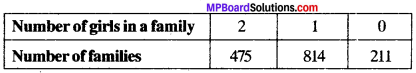Compute the probability of a family, chosen at random, having

1. 2 girls
2. 1 girl
3. No girl

Also check whether the sum of these probabilities is 1.
Solution:
Total number of families = 1500
Let E , E2 and E} denote the event that the families having 2 girls, 1 girl and no girl.
P(E1) = $$\frac{475}{1500}$$ = 0.317
P(E2) = $$\frac{814}{1500}$$ = 0.543
P(E3) = $$\frac{211}{1500}$$ = 0.140
P(E1) + P(E2) + P(E3) = 0.317 + 0.543 + 0.140 = 1.

Question 3.
In a particular section of Class IX, 40 students were asked about the months of their birth and the following graph was prepared for the data so obtained: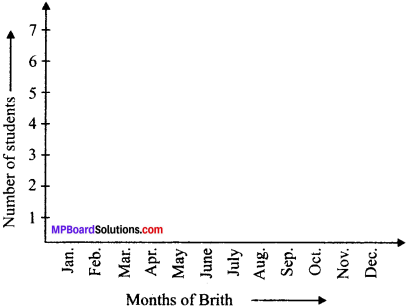Find the probability that a student of the class was born in August.
Solution:
Total number of students = 40
Number of students that were bom in the month of August = 6 Let E denotes the event that a student of the class was bom in August
P(E) = $$\frac{6}{40}$$ = 0.15

Question 4.
Three coins are tossed simultaneously 200 times with the following frequencies of different outcomes: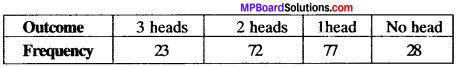If the three coins are tossed simultaneously tossed again, compute the probability of 2 heads coming up.
Solution:
Total number of trials = 200
Frequency of 2 heads coming up = 72
Let E denotes the event that 2 heads coming up
P(E) = $$\frac{72}{200}$$ = 0.36Question 5.
An organisation selected 2400 families at random and surveyed them to determine a relationship between income level and the number of vehicles in a family. The information gathered is listed in the table below: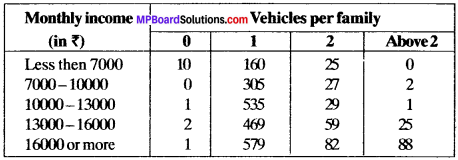Suppose a family is chosen. Find the probability that the family chosen is

1. earning ₹ 10000 -13000 per month and owning exactly 2 vehicles.
2. earning ₹ 16000 or more per month and owning exactly 1 vehicle.
3. earning less than ₹ 7000 per month and does not own any vehicle.
4. earning ₹ 13000 – 16000 per month and owning more then 2 vehicles.
5. owning not more than 1 vehicle.

Solution:
Total number of families = 2400

1. P (familiy chosen is earning ₹ 10000 – 13000 per month and owning exactly 2 vehicles)
= $$\frac{29}{2400}$$

2. P (that the family chosen is earning ₹ 16000 or more and owning exactly 1 vehicle)
= $$\frac{579}{2400}$$

3. P (that the family chosen is earning less than ₹ 7000 per month and does not own any vehicle)
= $$\frac{10}{2400}$$ = $$\frac{1}{240}$$

4. P (that the family chosen is earning ₹ 13000 – 16000 per month and owning more than 2 vehicles)
$$\frac{25}{2400}$$ = $$\frac{1}{96}$$

5. Number of families owning not more than 1 vehicle
= 10 + 1 + 2 + 1 + 160 + 305 + 535 + 469 + 579
= 2062
P (that the family chosen is owning not more than 1 vehicle)
$$\frac{2062}{2400}$$ = $$\frac{1031}{1200}$$

Question 6.
Refer the table below: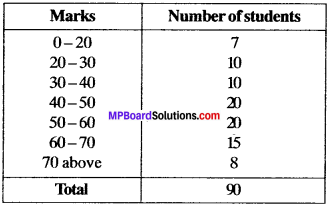1. Find the probability that a student obtained less then 20% in the mathematics test.
2. Find the probability that a student obtained marks 60 or above.

Solution:
Total number of students = 90

1. Number of students getting less that 20% marks = 7
Let E denotes the event th.-t a student obtained less than 20% in the mathematics test
P(E) = $$\frac{7}{90}$$ = 0.078

2. Number of students getting marks 60 or above = 15 + 8 = 23
Let E denote the event that a student obtained marks 60 or above.
P(E) = $$\frac{23}{90}$$ = 0.255

Question 7.
To know the opinion of the student about the subject statistics, a survey of200 students was conducted. The data is recorded in the following table.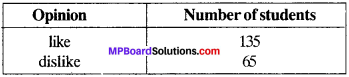Find the probability that a student chosen at random

1. likes statistics
2. does not like it.

Total number of students on which survey was conducted = 200
1. Let E1 denotes the event that the student chosen likes statistics.
P(E1) = $$\frac{135}{200}$$ = 0.675

2. Let E2 denotes the event that the student chosen dislikes statistics.
P(E2) = $$\frac{65}{200}$$ = 0.325Question 8.
The distance (in km) of 40 engineers from their residence to their place of work were found as follows: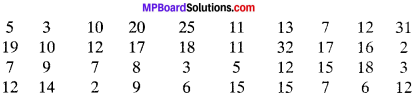What is the empirical probability that an engineer lives:

1. less than 7 km from her place of work?
2. more than or equal to 7 km from her place of work?
3. within 1/2 km from her place of work?

Solution:
1. Number of engineers residing less than 7 km from their place of work = 9
Total number of engineers = 40
Let E1 denotes the event that an engineer lives less than 7 km from her place of work.
P(E1) = $$\frac{9}{40}$$

2. Number of engineers residing more than or equal to 7 km from their place of work = 40 – 9 = 31
Let E2 denotes the event that an engineer lives more than or equal to 7 km from their place of work
P(E2) = $$\frac{31}{40}$$

3. Number of engineers residing within $$\frac{1}{2}$$ km = 0
Let E3 denotes the event that an engineer lives within $$\frac{1}{2}$$ km from their place of work.
P(E3) = $$\frac{0}{40}$$

Question 9.
Activity: Note the frequency of two-wheelers, three wheelers and four wheelers going past during a time interval, in front of your school gate. Find the probability that any one vehicle out of the total vehicles you have observed is a two-wheeler.
Solution:
Do if yourself.

Question 10.
Activity: Ask all the students in your class to write a 3-digit number. Choose any student from the room at random. What is the probability that the number written by her/him is divisible by 3? Remember that a number is divisible by 3, if the sum of its digits is divisible by 3.
Solution:
Do it with the help of your class mates.Question 11.
Eleven bags of wheat flour, each marked 5 kg, actually contained the following weights of flour (in kg);
4.97 5.05 5.08 5.03 5.00 5.06 5.08 4.98 5.04 5.07 5.00
Find the probability that any of these bags chosen at random contains more than 5 kg of flour.
Solution:
Total number of bags = 11
Number of bags containing more than 5 kg of flour = 7
Let E denotes the event that any of these bags chosen at random
contains more then 5 kg of flour.
p(E) = $$\frac{7}{11}$$

Question 12.
You were asked to prepare a frequency distribution table, regarding the concentration of sulphur dioxide in the air in parts per million of a certain city for 30 days. Using this table, find the probability of the concentration of sulphar dioxide in the interval 0.12 -0.16 on any of these days.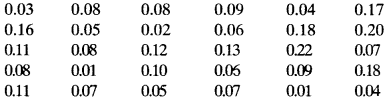Solution:
Frequency distribution table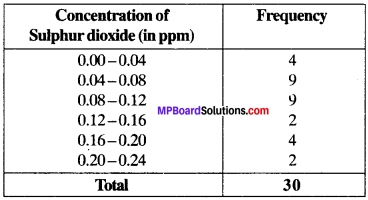Total number of days = 30
Let£ denotes the event of the concentration of sulphar dioxide in the interval 0.12 – 0.16 on any of these days.
p(E) = $$\frac{2}{30}$$ = $$\frac{1}{15}$$

Question 13.
You were asked to prepare a frequency distribution table regarding the blood groups of 30 students of a class. Use this table to determine the probability that a student of this class, selected at random, has blood group AS.
A, B, O, O, AB, O, A, O, B, A, O, B, A, O, O,
A, AB, O, A, A, O, O, AB, B, A, O, B, A, B, O.
Solution:
Frequency distribution table
Number of students having blood group AB = 3
Total number of students = 30
Let E denotes the event that a student of this class selected at random has blood group AB.
p(E) = $$\frac{3}{10}$$ = $$\frac{1}{10}$$